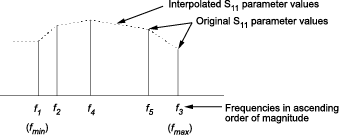# rfckt.passive

Passive component or network

## Description

Use the `passive` class to represent passive RF components and networks that are characterized by passive network parameter data.

Use the `read` method to read the passive object data from a Touchstone data file. When you read S-parameter data into an `rfckt.passive` object, the magnitude of your S21 data must be less than or equal to 1.

Due to random numerical error, data measured from a passive device is not necessarily passive. However, `rfckt.passive` objects can only contain passive data. To import data with active regions, use the `rfckt.amplifier` object, even if the original data represents a passive device.

## Creation

### Syntax

``h = rfckt.passive``
``h = rfckt.passive(Name,Value)``

### Description

example

````h = rfckt.passive` returns an passive-device object whose properties all have their default values.```
````h = rfckt.passive(Name,Value)` sets properties using one or more name-value pairs. For example, `rfckt.passive('IntpType','cubic')` creates an passive-device object with piecewise cubic Hermite interpolation as interpolation method. You can specify multiple name-value pairs. Enclose each property name in a quote. Properties not specified retain their default values.```

## Properties

expand all

Computed S-parameters, noise figure, OIP3, and group delay values, specified as `rfdata.data` object. ```Analyzed Result``` is a read-only property. For more information refer, Algorithms.

Data Types: `function_handle`

Interpolation method used in `rfckt.passive`, specified as a `1-by-N` character array of the following values:

MethodDescription
`Linear` (default)Linear interpolation
`Spline`Cubic spline interpolation
`Cubic`Piecewise cubic Hermite interpolation

Data Types: `char`

Object name, specified as an `1-by-N` character array. `Name` is a read-only property.

Data Types: `char`

Network parameter data, specified as a `rfdata.network` object.

Data Types: `function_handle`

Number of ports, specified as a positive integer. `nport`t is a read-only property. The default value is `2`.

Data Types: `double`

## Object Functions

 `analyze` Analyze RFCKT object in frequency domain `calculate` Calculate specified parameters for rfckt objects or rfdata objects `circle` Draw circles on Smith Chart `extract` Extract specified network parameters from rfckt object or data object `listformat` List valid formats for specified circuit object parameter `listparam` List valid parameters for specified circuit object `loglog` Plot specified circuit object parameters using log-log scale `plot` Plot circuit object parameters on X-Y plane `plotyy` Plot parameters of RF circuit or RF data on X-Y plane with two Y-axes `getop` Display operating conditions `polar` Plot specified object parameters on polar coordinates `semilogx` Plot RF circuit object parameters using log scale for x-axis `semilogy` Plot RF circuit object parameters using log scale for y-axis `smith` Plot circuit object parameters on Smith chart `write` Write RF data from circuit or data object to file `getz0` Calculate characteristic impedance of RFCKT transmission line object `read` Read RF data from file to new or existing circuit or data object `restore` Restore data to original frequencies `getop` Display operating conditions `groupdelay` Group delay of S-parameter object or RF filter object or RF Toolbox circuit object

## Examples

collapse all

Create passive RF components using `rfckt.passive`.

`pas = rfckt.passive('IntpType','cubic')`
```pas = rfckt.passive with properties: IntpType: 'Cubic' NetworkData: [1x1 rfdata.network] nPort: 2 AnalyzedResult: [1x1 rfdata.data] Name: 'Passive' ```

## Algorithms

The `analyze` method computes the `AnalyzedResult` property as follows:

The `analyze` method uses the data stored in the `'NetworkData'` property of the `rfckt.passive` object to calculate the S-parameter values of the passive component at the frequencies specified in `freq`. If the `'NetworkData'` property contains network Y- or Z-parameters, the `analyze` method first converts the parameters to S-parameters. Using the interpolation method you specify with the `'IntpType'` property, the `analyze` method interpolates the S-parameter values to determine their values at the specified frequencies.

Specifically, the `analyze` method orders the S-parameters according to the ascending order of their frequencies, fn. It then interpolates the S-parameters, using the MATLAB® `interp1` function. For example, the curve in the following diagram illustrates the result of interpolating the S11 parameters at five different frequencies.For more information, see “One-Dimensional Interpolation” and the `interp1` reference page in the MATLAB documentation.

As shown in the preceding diagram, the `analyze` method uses the parameter values at fmin, the minimum input frequency, for all frequencies smaller than fmin. It uses the parameters values at fmax, the maximum input frequency, for all frequencies greater than fmax. In both cases, the results may not be accurate, so you need to specify network parameter values over a range of frequencies that is wide enough to account for the component behavior.

The `analyze` method uses the S-parameters to calculate the group delay values at the frequencies specified in the `analyze` input argument `freq`, as described in the `analyze` reference page.

 EIA/IBIS Open Forum, Touchstone File Format Specification, Rev. 1.1, 2002